﻿ Internal Fluid Flow Induced Vibration of PipesPublications are Open
Access in this journal
Article Versions
Export Article
• Normal Style
• MLA Style
• APA Style
• Chicago Style
Research Article
Open Access Peer-reviewed

### Internal Fluid Flow Induced Vibration of Pipes

Etim S Udoetok
Journal of Mechanical Design and Vibration. 2018, 6(1), 1-8. DOI: 10.12691/jmdv-6-1-1
Published online: March 23, 2018

### Abstract

The flow induced vibration of pipes due to internal flowing fluid is studied and investigated. Equations were developed to express the influence of internal fluid flow on the natural frequency and displacement amplitude of vibrating pipes. The approach involved complex mechanics and geometric analysis to arrive at new and simple equations which compares favorably to experimental results. The proposed new equations can be widely used to check and limit flow induced vibration level. More light is shed on the phenomena of steady internal fluid flow exacerbating the vibration of pipelines and piping systems.

### 1. Introduction

Vibration of exposed pipe sections or systems due to external and/or internal fluid flow has been a common place in industrial plants and pipelines. It is common to use the vibration of piping systems as a quick check that a plant is operational by listening for the humming sound or by placing a hand on a pipe for the vibration feel. The source(s) of vibrations of a piping system could be any or a combination of the following:

(i) Pumps

(ii) Valves

(iii) Strainers

(iv) Fans

(v) Pressure fluctuations

(vi) Weak foundation and support structures

(vii) Externa cross-flows

(viii) Internal fluid flow

Severe or unusual high vibration of piping systems could be a sign of imminent failure of pipe, imminent failure of devices such as strainers, valves, pumps, etc., or due to a loose and semi-heavy machine part or tool in the pipeline. The vibration level, if unchecked, can adversely affect the life span of a piping system 1, 2, and it can consequently affect the operational capacity and life span of the plant which it is a part of. Therefore, it is important to understand vibration in the case of internal flow in pipes.

Early work on flow induced vibrations dates back to the 1940s when above-ground oil pipelines operated by Trans-Arabian Pipeline Company were observed to have a complex vibration characteristic due to internal fluid flow especially when perturbed by external cross winds 3, 4. Early theoretical works were based on the bending vibration of a beam on simple supports 3, 4, 5. The nuclear energy industry has found works on flow induced vibration very useful and some countries have developed different allowable vibration criteria to prevent failure 2, 6, 7. The usual initial response to check vibration is slowing down the system and checking the supports but this result in reducing system capacity. Other methods have been investigated and this includes making flow rate lower than a defined critical velocity 5, 8, varying elasticity of supports 9. The effect of core-annular fluid flow was also investigated 10 and even the effect of concentrated masses on flow induced vibration 11. The minimization or elimination of flow induced vibration would be easier if the phenomenon is well understood. Works on flow induced vibrations have involved experimentation 12, 13, and computer aided simulations 14, 15. However, the information on flow vibration is seldom complete 16. Therefore, the work presented in this paper tends to develop a more accurate theoretical analysis and recommends best approaches to reduce or eliminate flow induced vibrations summarized from published works and experience.

### 2. Method

2.1. Analysis of Flow Induced Vibration of a Pipe with Clamped Supports

In this section, flow induced will be analyzed for cases typically encountered in plants and petroleum pipelines where the piping system or pipeline section is designed to be structurally strong. Therefore, analysis will be done with the assumptions that supports are rigid clamps, vibration amplitude is much less than the free length of the pipe, and vibrating section is strong enough to resist transitioning into the second mode vibration. Consider a horizontal pipeline section clamped with rigid supports at both ends. The whole pipe section has homogeneous cross-section. Fluid is flowing through at an average velocity, V (see Figure 1). Assuming an initial force perturbs the pipe downward at the midpoint and it then vibrates at its natural frequency with the internal fluid flow. In Figure 1, Fflex is the flexural reaction and Fcen is the centripetal force due the effect of the curvature of the vibrating pipe section on the internal fluid flow. Summation of forces in the radial direction on the elemental fluid element in the pipe is given as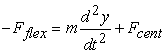(1)

where m is the total mass of the pipe and fluid per unit length, and y is the deflection at location x along the pipe length. Moving all the terms to one side gives(2)
• Figure 1. Schematic of vibrating pipe section

From mechanics 17, 18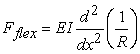(3)

and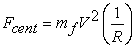(4)

where R is the radius of curvature and EI is the flexural stiffness. Equations (3) and (4) into Equation (2) gives(5)

where mf is the fluid mass per unit length, and mtot is the fluid plus pipe mass per unit length. All the turbulence effect and pipe-fluid friction is captured in the value of V. From mechanics 17(6)

Equation (6) into (5) gives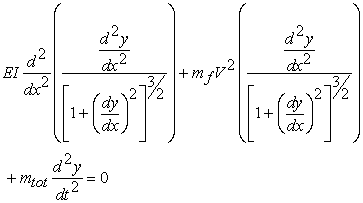(7)

Recall the assumption that pipe section is structurally strong such that deflection is small, so higher orders of the slope,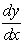, can be neglect to arrive at a simplified form of Equation (7)(8)

subject to the boundary conditions (BCs)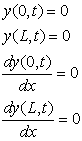(9)

and initial condition(10)

Assuming a solution of the form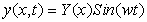(11)

And assume solution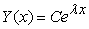yields a direct solution of the form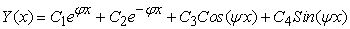(12)

whereandand C1, C2, C3 and C4 are integration constants. The direct solution, Equation (12), is complex to work with, and the application of BCs (Equation (9)) results in a trivial solution due to the error in the assumption made to arrive at the governing equation, Equation (8), and due to the nature of the BCs. Assuming a polynomial solution of the form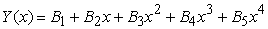(13)

which is a viable solution since the direct is an exponential function which can be expanded into a polynomial by series expansion. Applying BCs to Equation (13) gives the following:The solution is now(14)

where B5 and w are yet to be found. Equation (14) into Equation (8), would yield w has a function of x.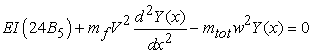(15)

Therefore, average values of centripetal force and average value of deflection would be used to eliminate x in Equation (15).(16)

The peak value of deflection occurs at the middle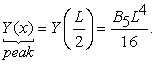(17)

Finding the average value of the centripetal force requires the aid of following the fluid path in a sketch as shown in Figure 2. With the assumption of negligible slope, Equation (6) can be written as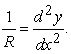(18)

Therefore the curvature varies with x and changes direction twice along the deflected pipe. The points at which the directions changes have zero centripetal forces and are found by equating Equation (18) to zero.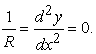(19)

Combining Equations (19) and (14) gives(20)

Average inverse of curvature peaks in the different zones are given as

for x = 0 to x1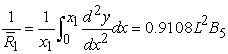(21)

for x = x1 to L/2(22)

From geometric relations for a secant, in the region of x = 0 to x1 the inverse of curvature can be approximated as 19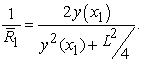(23)

Combining Equations (14), (20), (21) and (23) gives(24)

where the peak values of the sine function has been used

Equation (24) gives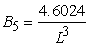(25)

where the positive value has been taken in line with the direction of y. And this value of B5 is only an estimate local to the regions x = 0 to x1 and x2 to L. The value of θ can now be calculated from the geometric relation 19,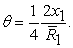(26)

Equations (20), (21) and (25) into (26) gives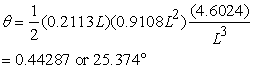• Figure 2. Schematic showing the two set of centripetal forces and the lines of their resultant force

Since V is the same everywhere along x (mass is conserved and flow is incompressible), the overall centripetal force is estimated as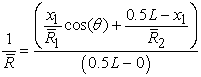(27)

Equations (20),(21) and (22) into Equation (27) gives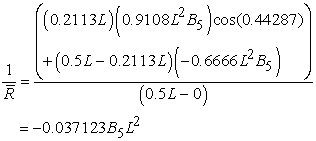(28)

where symmetry and discontinuity have been taken into account. The sign of the overall inverse of curvature shows that the bending effect of the central curvature dominates. Therefore, Equations (28) and (17) into (16) gives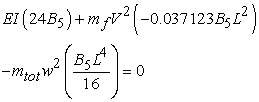(29)

which implies that(30)

Equation (30) gives the natural frequency for the case of clamped (fixed) ends of the vibrating pipe section. In order to estimate B5, mechanics method for analyzing suddenly applied load is applied. Assume that the deflection of the pipe at the middle of the pipe free length is modeled as a spring (see Figure 3), the spring constant, k, is estimated from the equation for deflection under static load as 17, 18(31)

for clamped end and uniformly distributed load. The governing equation for this case is(32)

where ym is the defection at the middle of the vibrating pipe and the second term on the right hand side account for the centripetal force due to the curvature of the pipe. Equation (32) is subject to the initial condition(33)

where u is the maximum or peak velocity at y = 0.

• Figure 3. Mass-spring model of the deflection at the middle of the vibrating pipe of Figure 1

Neglecting 2nd and higher orders of ym in line with the assumption that the deflection is much smaller than the pipe length, Equation (32) simplifies to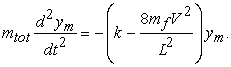(34)

Solving Equation (34) gives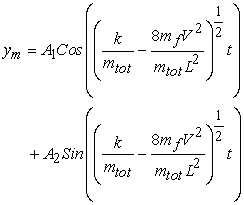(35)

Applying the initial conditions (Equation (33)) gives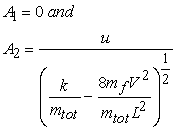(36)

Equation (36) into Equation (35) gives the solution as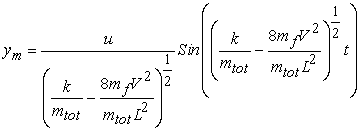(37)

Therefore, the peak value gives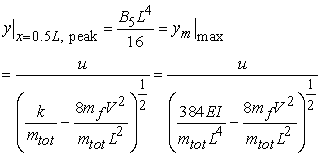(38)

which implies that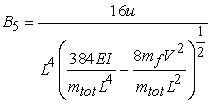(39)

and the solution to the vibrating pipe becomes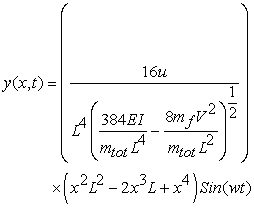(40)

The peak velocity, u, in Equation (40) can be easily measured with a vibration sensor.

While simple supports are not recommendable, brief analyses of such a case is done for comparison in the next subsection.

2.2. Flow Induced Vibration of Pipe on Simple Support

Consider a case similar to that analyzed above, but with pipe ends simply supported (see Figure 4). Also assuming that the pipe is strong enough to resist big slope and resist transition to second mode vibration. The governing equation will remain the same but BCs will change as given below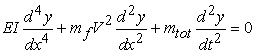(8)

subject to the boundary conditions (BCs)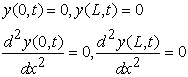(41)

and initial condition(10)

Since there is no moment at pipe ends, the curvature of the pipe is in the same direction throughout the pipe length and zeros out at the ends of the free section of the pipe i.e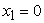and(see Equation 41). Therefore, a trigonometric function, which is a simpler function, can be fitted instead of the polynomial function. Assuming a trigonometric solution of the form(11)

and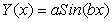(42)

Applying the BCs from Equation (41), it is clear that the first and third BCs are satisfied by the sine function. The second and fourth BCs both give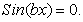(43)

Therefore,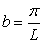(44)

where first mode solution is used.

• Figure 4. Schematic of vibrating pipe section for the case of simple supports

Equation (42) can now be expressed as(45)

Equations (45) and (11) into Equation (8) gives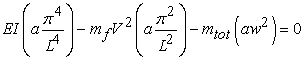(46)

The sine function easily canceled out on both sides to leave the peak values and average inverse of curvature. Equation (46) implies that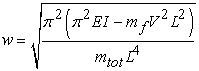(47)

In order to estimate a, mechanics method similar to that used for estimating B5 is applied. For this case, the spring constant, k, is estimated from the equation for deflection under static load as 17, 18(48)

for simply supported and uniformly distributed load. The governing equation for this case is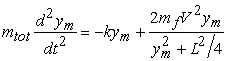(49)

where ym is the defection at the middle of the vibrating pipe and the second term on the right hand side account for the centripetal force due to the curvature of the pipe. Equation (49) is subject to the initial condition(40)

where u is the maximum or peak velocity at y = 0.

Neglecting 2nd and higher orders of ym in line with the assumption that the deflection is much smaller than the pipe length, Equation (49) simplifies to(51)

Solving Equation (51) gives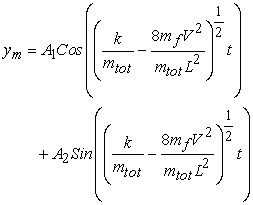(52)

Applying the initial conditions (Equation (50)) gives(53)

Equation (53) into Equation (52) gives the solution as(54)

Therefore, the peak value gives(55)

and the solution to the vibrating pipe becomes(56)

The peak velocity, u, in Equation (56) can also be easily measured with a vibration sensor.

2.3. Critical Velocity for the Two Cases Analyzed

Critical velocity is of great importance in flow induced vibration and is defined as the velocity at which the natural frequency is zero (w = 0). Beyond the critical velocity instability takes over and the pipe vibrates critically. The critical velocities for the cases analyzed are estimated from Equations (30) and (47). w = 0 gives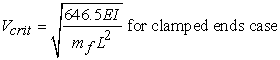(57)

and(58)
2.4. Stress due to Vibration

The dynamic stress, σ, resulting from the flow induced vibration can be expressed as 17, 20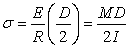(59)

whereand D is the external diameter of the pipe. The maximum stress occurs at the middle of the free length of the pipe in line with the first mode vibration, therefore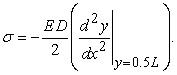(60)

The maximum stresses for the cases analyzed are estimated by combining Equations (40) and (56) with Equation (60) using peak values(61)

and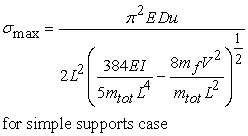(62)

### 3. Comparison with Published Experimental Results and Other Models

Most published works reviewed were based on simple supports, so few were found that focus on clamped (fixed) ends. Zhang et al 13 provided enough information on their experimental work on clamped, so the proposed new method, Equation (30), was compared with the first mode results published and comparison is shown in Table 1.

The published experimental work used to compare with the simple support case is that of Dodds et al 5. The published experimental work of Dodds et al is compared with Equation (47) and shown in Table 2. They used two pipes of same material and dimensions.

The critical velocity accuracy simply follows the accuracy of the natural frequency equations. Zhang et al 13 did not experimentally measure the critical velocity, but Dodds et al did for the aluminum pipe. The critical velocity they obtained experimentally by Dodds et al was 38.862 m/s while that obtained using the proposed model, Equation (58), is 40.818 m/s. Peak vibration velocities, u, were not record by either Zhang et al nor Dodds et al. Peak transverse velocity reading, u, measured at the middle of pipe free length using a vibration sensor (which is also referred to as a vibration meter or vibrometer) is required in order to evaluate peak deflections and maximum stress using the proposed new model. However, it is recommended that u be less than 12.7mm/s to avoid failure 1, 6, 7 especially in nuclear power plants.

### 4. Discussion

The new method used to analyze flow induced vibration involved properly accounting for the effect of centripetal force due to the pipe’s deflection. All the internal flow effects are captured in the average velocity value, V. The average velocity, V, was assumed to be constant which means that the result won’t be accurate for flows with pulsation. The new method eliminates the need for extensive complex analysis like the work of Semler et al 21.

The equations for the natural frequencies of the flow induced vibration of the pipe obtained shows that the natural frequency reduces as the flow tends towards the critical velocity. For the same pipe and flow conditions, the clamped ends case’s natural frequency starts slightly higher than that of the simply supported ends case as the fluid velocity is increased from zero (see Equations (30) and (47)). But the simply supported ends case’s natural frequency quickly approaches zero where the critical velocity occurs. Therefore, the clamped ends case is more stable for a wider range on velocity and hence fully recommended for safety and for prevention of failure. The new natural frequency equations, Equations (30) and (47), compared favorably with experimental data as shown in Table 1 and Table 2, though there was negligible difference probably due to experimental error and approximations.

The development of solution s to the vibration problems as given by Equations (40) and (56) is unprecedented so no model or experimental data were found for comparison. However, energy conservation method applied to the mass-spring model of the mid-point deflection will arrive at the same solution.

The deflection solution provided by Equations (40) and (56) shows that peak deflection increases with increase in flow velocity even while frequency is reducing. This increased vibration amplitude exacerbates the vibration of the pipe as the velocity is increased. The deflection solution is also vital to finding the maximum stress expressed in Equations (61) and (62). Additionally, the recommended transverse vibration peak velocity value (u < 12.7 mm/s 1, 6, 7) can be used to check designs and recommend solutions by combination with deflection equation or maximum stress equation.

The new critical velocities developed, Equations (57) and (58), clearly shows that the critical velocity is a function of the fluid mass per unit length and not the total (fluid + pipe) mass per unit length as presented by other authors. Therefore, for very thick pipes I is higher and critical velocity is increased which implies that the system will resist or tolerate higher velocities according to Equations (57) and (58), but wrongly replacing the fluid mass per unit length with the total mass per unit length for a thick pipe will predict a much lower critical velocity than the right value.

The new analysis and model for flow induced vibration presented are useful tools for tackling vibration problems. For example, putting new supports at the middle a free pipe length, automatically halves the value of L and reduces the vibration level. Pipe supports should be rigid and connected to strong foundations for proposed new model to be applicable. Buried pipe sections should have sufficient cover and where the cover soil is quick or loose, intermittent rigid supports or compact soil should be used. Valves mounted on supports should be preferred. In a nut shell, vibrations from other sources should be checked when tackling flow induced vibration problem.

### 5. Conclusion

Flow induced vibration model has been developed for the case of clamped ends of a free pipe section and the case of simply supported ends of a free pipe section. The development method involved mechanics and geometric analysis. Expressions were developed for natural frequency, pipe deflection, critical velocity and maximum stress due to vibration. Results were compared with published experimental results and found to be in good agreement.

### Acknowledgements

The author acknowledges the help of Mr Edidiong Nseowo Udofia in finding some of the reference materials used to check this work.

### References

  Fomin, V.V., Kostarev, V.V., and Reinsch, K-H. “Elimination of Chernobyl NPP Unit 3 Power Output Limitation Associated with High Main Steam Piping Flow Induced Vibration.” Transactions of the SMiRT 16, Washington DC, August 2001. In article View Article  Blevins, R.D. “Flow-Induced Vibration in Nuclear Reactors, a Review,” Progress in Nuclear Energy Vol. 4, pp 25-49, 1979. In article View Article  Ashley, H., and Haviland, G. “Bending Vibrations of a Pipe Line Containing Flowing Fluid,” Journal of Applied Mechanics, 72, pp 229-232, 1950. In article  Den Hartog, J.P. Mechanical Vibrations, McGraw-Hill, New York, 1940, ch. 7. In article  Dodds, H.L., and Runyan, H. “Effect of High-Velocity Fluid Flow in the Bending Vibrations and Static Divergence of a Simply Supported Pipe.” National Aeronautics and Space Administration Report NASA TN D-2870, June 1965. In article  Xue, F., Lin, L., Ti., and W. Lu, N. “Vibration Assesment Method and Engineering Application to Nuclear Power Plants,” International Atomic Energy Agency’s 2nd International Symposium on Nuclear Power Plant Life Management, Shangai, China, October 2007. In article  Caillaud, S., Briand, D, Moussou, and P., Gaudin, M. “Correction Factors for ASME ANSI-OM-3 Stress/Velocity Relationship With Respect to Static Design,” Transactions of the 17th International Conference on Structural Mechanics in Reactor Technology (SMiRT 17) Prague, Czech Republic, August, 2003. In article  Czerwinski, A, and Luczko, J. “Vibration of Steel Pipes and Flexible Hoses Induced by Periodically Variable Fluid Flow,” Mechanics and Control, Vol. 31, No. 2, pp 63-71, 2012. In article View Article  Faal, R.T., and Derakhshan, D. “Flow-Induced Vibration of Pipeline on Elastic Support,” Procedia Engineering, Vol. 14, pp 2986-2993, 2011. In article View Article  Gorman, D.G., Liu, M., and Horacek, J. “The Effect of Annular Two-Phase Flow upon the Natural Frequencies of a Pipe,” Engineering Structures, Vol. 20, No. 8, pp 726-731, 1998. In article View Article  Kang, M-G. “Effect of Rotary Inertia of Concentrated Masses on the Natural Vibration of Fluid Conveying Pipes,” Journal of Korean Nuclear Society, Vol. 31, No. 2, pp 202-213, 1999. In article View Article  Thompson, A.S. “Experimental Characterization of Flow Induced Vibration in Turbulent Pipe Flow,” Master’s Thesis, Brigham Young University, Utah-USA, 2009. In article View Article  Zhang, Y.L., Gorman, D.G, and Reese, J.M. “Analysis of the Vibration of Pipes Conveying Fluid,” Proc. Instn. Mech. Engrs., Vol. 213, pp 849-860, 1999. In article View Article  Veerapandi, R., Karthikeyan, G., Jinu, G.R., and Kannaiah, R., “Experimental Study and Analysis of Flow Induced Vibration in a Pipeline,” International Journal of Engineering Research and Technology, Vol. 3, No. 5, pp 1996-1999, 2014. In article  Vasilyev, P., and Fromzel, L. “Analytical Study of Piping Flow-Induced Vibration. Example of Implementation,” Transactions of the 17th International Conference on Structural Mechanics in Reactor Technology (SMiRT 17) Prague, Czech Republic, August, 2003. In article View Article  Paidoussis, M.P. “Real-Life Experiences with Flow-Induced Vibration,” Journal of Fluids and Structures, Vol. 22, pp 741-755, 2006. In article View Article  Shigley, J.E., and Mischke, C.R. Mechanical Engineering Design, 6th Ed., McGraw-Hill, New York 2001, pp 178, 179, 1182, 1186. In article  Spiegel, L., and Limbrunner, G.F. Applied Statics and Strength of Materials, 2nd Ed., Prentice Hall, New Jersey 1993, pp 359-371, 727, 730. In article View Article  Harris, J.W., and Stocker, H. “Segment of a Circle.” In Handbook of Mathematics and Computational Science, Springer-Verlag, New York, 1998, pp 92-93. In article  Drozyner, P. “Determining the Limits of Piping Vibrations.” Scientific Problems of Machines Operation and Maintenance, Issue 165, No. 1, pp 97-103, 2011. In article View Article  Semler, C, Li, G.X, and Paidoussis,M.P. “The Non-Linear Equations of Motion of Pipes Conveying Fluid.” Journal of Sound and Vibration, Vol. 169, No. 5, pp 577-599, 1994. In article View ArticleThis work is licensed under a Creative Commons Attribution 4.0 International License. To view a copy of this license, visit http://creativecommons.org/licenses/by/4.0/